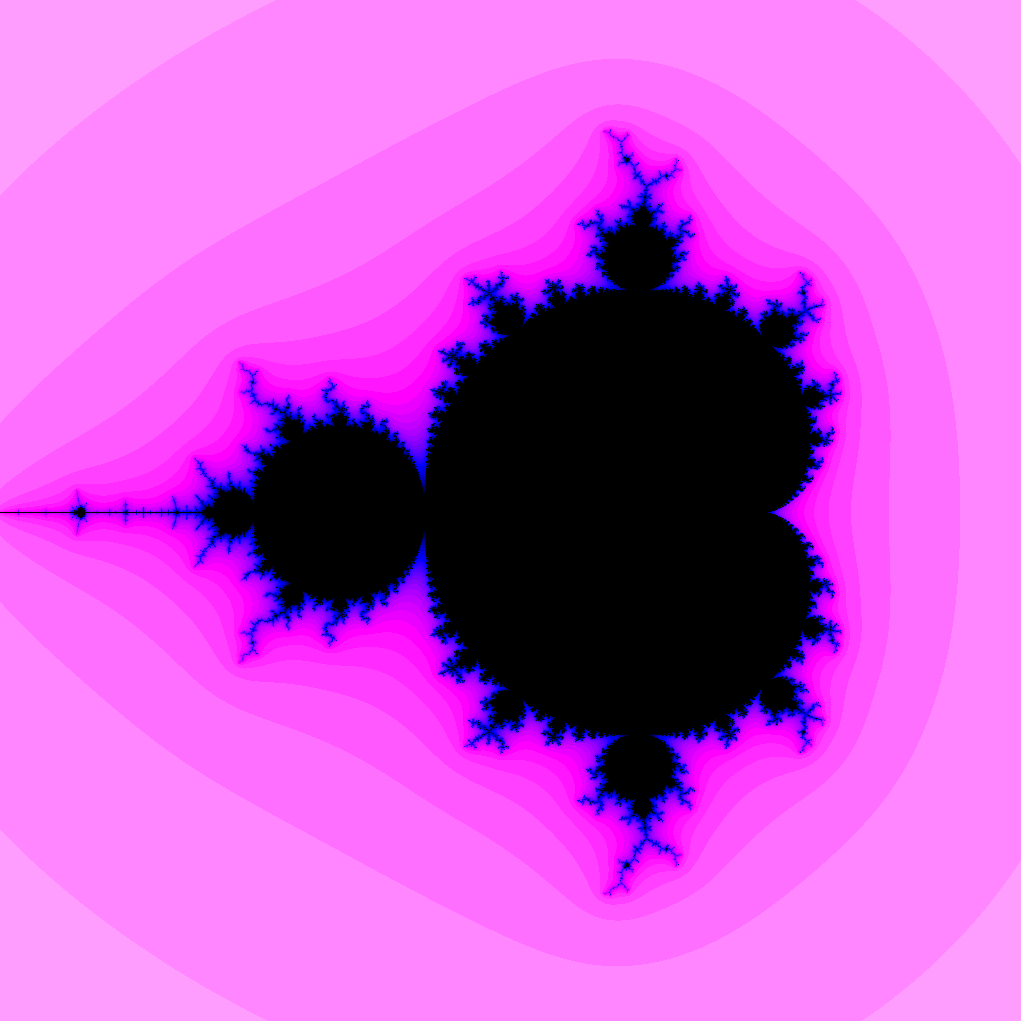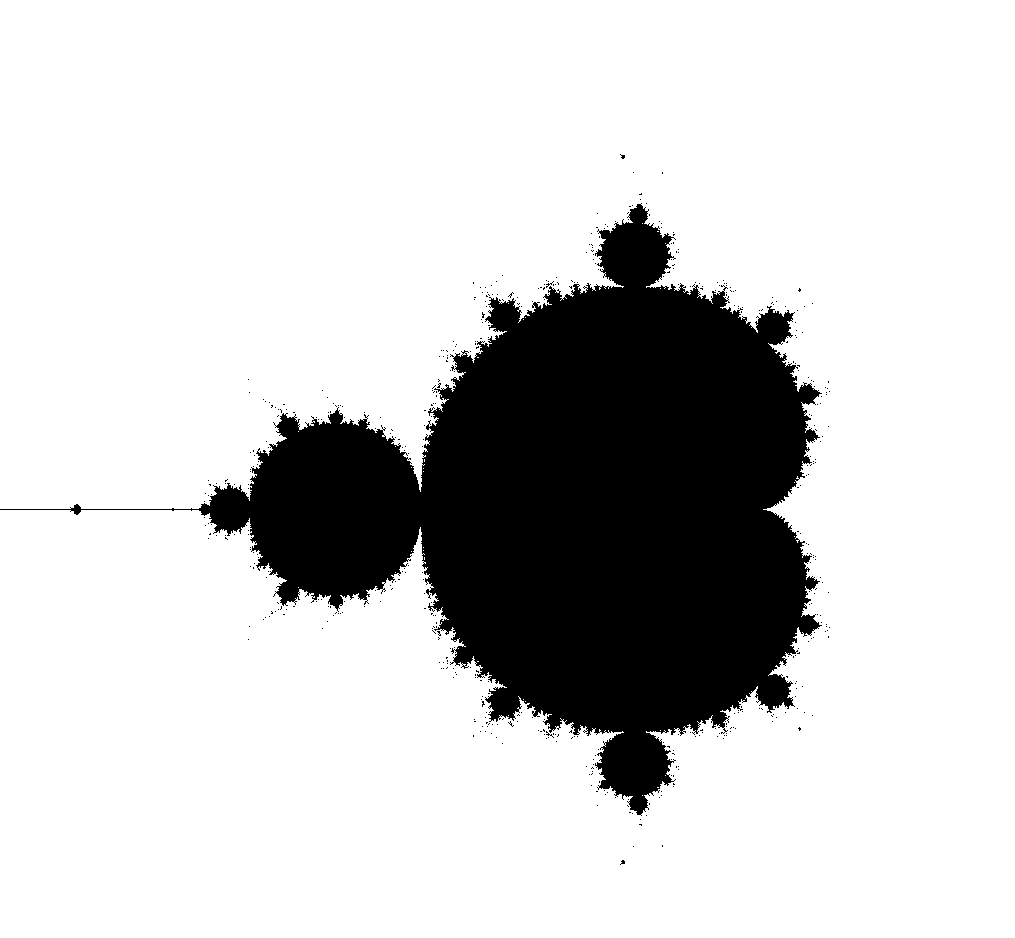DUE: Friday, March 26, 23:59 AoE#### Background

##### The Mandelbrot set

The Mandelbrot set is among the most widely depicted mathematical objects. In order to define the Mandelbrot set (and understand how to draw it), we need to start with the complex numbers and the complex plane.

###### Complex numbers

Complex numbers are numbers of the form $$a + b i$$ where $$a$$ and $$b$$ are real numbers, and $$i$$ is the imaginary number satisfying $$i^2 = -1$$. Complex numbers can be added and multiplied:

• $(a + b i) + (c + d i) = (a + c) + (b + d)i$
• $(a + b i) (c + d i) = (a c - b d) + (a d + b c) i$

Further, complex numbers have a modulus defined by

• $| a + b i | = \sqrt{a^2 + b^2}$

The complex plane consists of the set of all complex numbers represented as points in a plane: each $$a + b i$$ corresponds to the point $$(a, b)$$ in the plane.

###### Mandelbrot definition

Given a complex number $$c = a + b i$$, consider the sequence of complex numbers $$z_1, z_2, \ldots$$ defined by the following recursive formula:

• $z_1 = c$
• for $$n > 1$$, $$z_n = z_{n-1}^2 + c$$.

For each starting point $$c$$, one of two things can happen:

1. the sequence $$z_1, z_2, \ldots$$ remains bounded—i.e., there is a positive (real) number $$M$$ such that $$\mid z_n \mid \leq M$$
2. the sequence $$z_1, z_2, \ldots$$ is unbounded—$$\mid z_1 \mid , \mid z_2 \mid , \ldots$$ grows without bound.

The Mandelbrot set is defined to be the set of complex numbers $$c$$ for which the sequence $$z_1, z_2, \ldots$$ remains bounded. For example, $$c = 1$$ is not in the Mandelbrot set because its corresponding sequence is $$1, 2, 5, 26, \ldots$$ which grows without bound. On the other hand, $$c = -1$$ is in the Mandelbrot set because its corresponding sequence is $$-1, 0, -1, 0, \ldots$$ which remains bounded.

###### Computing the Mandelbrot set

In general, it might be difficult to determine if a given sequence $$z_1, z_2,\ldots$$ remains bounded analytically. To visualize the Mandelbrot set it is enough to determine if the sequence is bounded empirically. Specifically, we can choose two parameters: a modulus bound $$M$$ and an iteration bound $$N$$. We then proceed as follows. Starting from a complex number $$c$$, compute $$z_1, z_2, \ldots$$ as required. For each $$z_n$$, compute $$\mid z_n\mid$$ and do the following:

1. if $$\mid z_n\mid \geq M$$, stop and report that $$c$$ is not in the Mandelbrot set,
2. if $$n = N$$, stop and report that $$c$$ is in the Mandelbrot set
3. otherwise, continue.

Following this procedure, we can obtain this following image of the Mandelbrot set.For points $$c$$ not in the Mandelbrot set (i.e., for which case 1 above occurs), the first iteration $$n$$ at which $$\mid z_n \mid \geq M$$ is called the escape time. In order to generate a color image such as the image below, the color is determined by the escape time.Specifically, the color of a point $$c$$ is determined by a color map applied to the escape time for the point. In the image above, brighter colors indicate shorter escape times. You can read about different methods for coloring the Mandelbrot set at the following link:

##### The executor framework

The executor framework in Java provides a robust structure for handling tasks, where each task is specified as an instance of class implementing the Runnable (or Callable) interface. For this lab, you should use a “fixed thread pool” to execute tasks in parallel. This can be done by using the following the code, where class MyTask implements Runnable:

1
2
3
4
5
6
7
8
9
10
11
12
13
14
15
16

// create and execute a task

// shutdown the pool and wait for all pending tasks to complete
pool.shutdown();
try {
pool.awaitTermination(Long.MAX_VALUE, TimeUnit.NANOSECONDS);
} catch (InterruptedException e) {
// do nothing
}


Here are links to the relevant documentation:

#### Program description

For your assignment, you should write a program that computes and draws the Mandelbrot set. Your program must use the executor framework above in order to parallelize its computation.

Specifically, you should complete an implementation of the MandelbrotFrame class linked to below. The most computationally intensive method that you must implement is void updateEscapeTimes(). For this method, you should apply multithreading using the aforementioned executor service. You might consider making a task consisting of computing esc[i][j] for a single pair of values i, j.

#### What to turn in

To complete the assignment, turn in your completed MandelbrotFrame class, together with any other files needed for your implementation. Be sure to add a more interesting color map as well. (See Color documentation and Plotting algorithms for the Mandelbrot set)

To test your code’s correctness, use the provided StaticViewer program and compare it to the graphical output above. You will also be provided with a benchmarking program to test your code’s performance.

Your programs will be graded on a 6 point scale according to the following criteria:

• Correctness (2 pts). Your program compiles and completes the task as specified in the program description above.

• Style (2 pt).
• Coding style: code is reasonably well-organized and readable (to a human). Variables, methods, and classes have sensible, descriptive names. Code is well-commented.
• Output style: color map makes a visually appealing image.
• Performance (2 pts). Performance of updateEscapeTimes() method is comparable to the instructor’s implementation.

#### Extensions

There are a lot of directions you can go with this assignment! Here are a few:

1. Make a color map that makes things look much better than mine, and use it to make some nice images. (You can submit image files if you’d like.)
2. Modify the StaticViewer to do an infinite zoom into an interesting portion of Mandelbrot set. If you do this, have the program print the framerate to the console.
3. Draw other fractals! The Mandelbrot set is but one fractal in a large family of fractals produced using similar methods. For example, you could draw Newton fractals or other Julia sets.